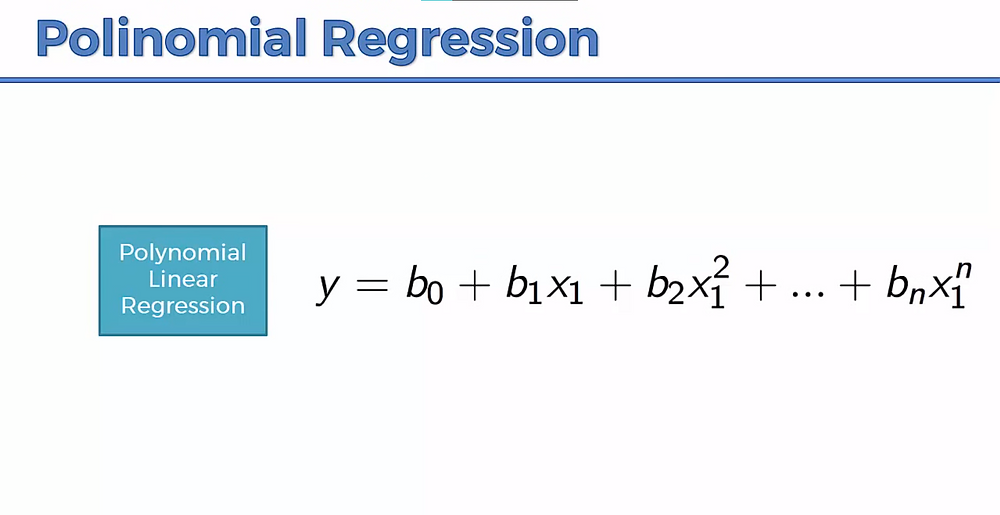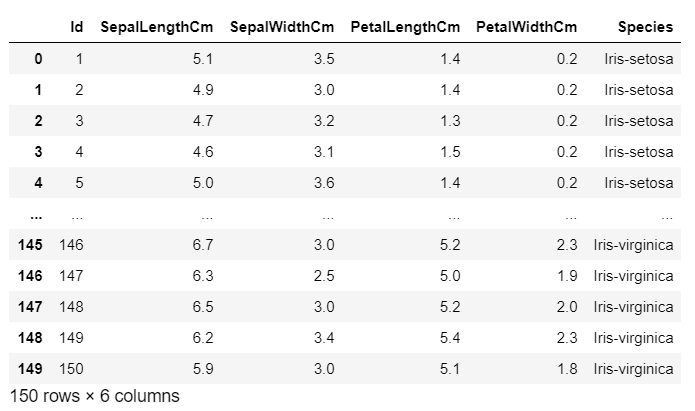Search

# Polynomial Linear Regression : Explained with an example.

There are many types of Linear regression in which there are Simple Linear regression, Multiple Regression, and Polynomial Linear Regression.The polynomial regression is similar to multiple regression but at the same time, instead of different variables like X1, X2, ..... Xn, we have the same variable X1 but it is in different power. So instead of X2 we have, X1^2, instead of X3 we have x1^2 and so on. So basically we are using one variable but we are using different power of that variable.

When we should use polynomial regression?

Let's say we have an observation like the below image, then the line that fits this data is obviously a simple linear regression. you can see that data fits really a well.But imagine, if your data set is like this. So if you try using simple linear regression, you can see that few data lying below the line.So how can we correct them? We can try to correct that using polynomial which is in this case fits perfectly. and you can see the formula also in the below image. in the simple regression, we are adding b2x1^2 which is giving us a parabolic effect.Polynomial regression is a bit different than simple regression but at the same time, it has its different use cases that come on a case by case. You have to apply simple regression, multiple regression and polynomial regression and see what happens. At some point, polynomial regression fits better. For e.g. there is a use to describe hoe the diseases are spreading and how the pandemics are spreading across the territory, across the globe.

Question: Why is it called linear still?

The trick here is that when we are talking about linear regression, we are not talking about x variable. even there is a nonlinear relationship between y and x, When we are talking about the class of regression, we are talking about the coefficient b. here be is in linear that is b, b1, b2, ... bn.So here we are finding the value of the coefficient i.e. b and then we are simply plugin x and find out the value of y. That's why linear and nonlinear regression refers to the coefficient. Polynomial regression is also called a special case of multiple regression.

Now we will see how to apply polynomial regression with python.

Here we are taking the iris dataset.

```import numpy as np
import pandas as pd
X = dataset.iloc[:, [1,2]].values
y = dataset.iloc[:, -1].values

dataset``````from sklearn.model_selection import train_test_split
X_train, X_test, y_train, y_test = train_test_split(X, y, test_size = 0.2, random_state = 1)

from sklearn.preprocessing import StandardScaler
sc = StandardScaler()
X_train= sc.fit_transform(X_train)
X_test = sc.transform(X_test)```

```from sklearn.svm import SVC
classifier = SVC(kernel = 'poly',random_state = 0)
classifier.fit(X_train, y_train)```

Output: SVC(kernel='poly', random_state=0)

```ypred = classifier.predict(X_test)
print ((ypred))``````y_pred = classifier.predict(X_test)
print(np.concatenate((y_pred.reshape(len(y_pred),1), y_test.reshape(len(y_test),1)),1))``````from sklearn.metrics import confusion_matrix, accuracy_score
cm = confusion_matrix(y_test, y_pred)
print(cm)
accuracy_score(y_test, y_pred)```

Output: [[11 0 0] [ 0 1 12] [ 0 0 6]]

Accuracy: 0.6

In statistics, polynomial regression is a form of regression analysis in which the relationship between the independent variable x and the dependent variable y is modeled as an nth degree polynomial in x. Polynomial regression fits a nonlinear relationship between the value of x and the corresponding conditional mean of y, denoted E(y |x), and has been used to describe nonlinear phenomena such as the growth rate of tissues, the distribution of carbon isotopes in lake sediments, and the progression of disease epidemics. Although polynomial regression fits a nonlinear model to the data, as a statistical estimation problem it is linear, in the sense that the regression function E(y | x) is linear in the unknown parameters that are estimated from the data. For this reason, polynomial regression is considered to be a special case of multiple linear regression.

See All